## 8240819346

##### EMAIL:
###### info@mcatutorials.com
Enroll now!
 Name Cources BTECH MCA MSC BCA BSC Others -- Choose Your Subject -- Computer Organization C Language Data Structure Digital Electronics Object Oriented Programming Discrete Mathematics Graph Theory Operating Systems Software Engineering Computer Graphics Database Management System Operation Research Computer Networking Image Processing Internet Technologies Micro Processor Artificial Intelligence Soft Computing E-Commerce & ERP C ++ Language C Programming C Data Structure Using C, C ++ Sql & PL/Sql Visual Basic Unix and Shell programming Java J2EE Oracle 9i 8085 Programming Android Application Development i-phone Application Development JAVA Swing based applications PHP, MySql, Ajax ASP.net Visual Basic Client Server Applications Email Phone# Loop

There may be a situation, when you need to execute a block of code several number of times. In general, statements are executed sequentially: The first statement in a function is executed first, followed by the second, and so on.

Programming languages provide various control structures that allow for more complicated execution paths.

A loop statement allows us to execute a statement or group of statements multiple times and following is the general form of a loop statement in most of the programming languages: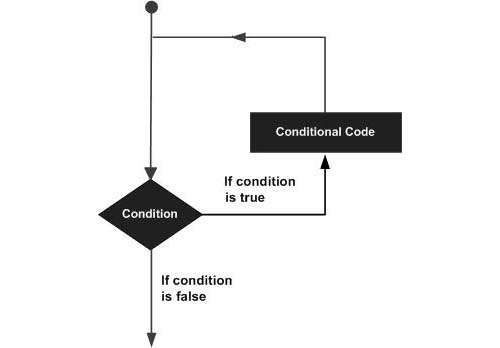C programming language provides the following types of loop to handle looping requirements. Click the following links to check their detail.

Loop TypeDescription
while loopRepeats a statement or group of statements while a given condition is true. It tests the condition before executing the loop body.
for loopExecute a sequence of statements multiple times and abbreviates the code that manages the loop variable.
do...while loopLike a while statement, except that it tests the condition at the end of the loop body
nested loopsYou can use one or more loop inside any another while, for or do..while loop.

## Loop Control Statements:

Loop control statements change execution from its normal sequence. When execution leaves a scope, all automatic objects that were created in that scope are destroyed.

C supports the following control statements. Click the following links to check their detail.

Control StatementDescription
break statementTerminates the loop or switch statement and transfers execution to the statement immediately following the loop or switch.
continue statementCauses the loop to skip the remainder of its body and immediately retest its condition prior to reiterating.
goto statementTransfers control to the labeled statement. Though it is not advised to use goto statement in your program.

## The Infinite Loop:

A loop becomes infinite loop if a condition never becomes false. The for loop is traditionally used for this purpose. Since none of the three expressions that form the for loop are required, you can make an endless loop by leaving the conditional expression empty.

```#include <stdio.h>

int main ()
{

for( ; ; )
{
printf("This loop will run forever.\n");
}

return 0;
}
```

When the conditional expression is absent, it is assumed to be true. You may have an initialization and increment expression, but C programmers more commonly use the for(;;) construct to signify an infinite loop.

A while loop statement in C programming language repeatedly executes a target statement as long as a given condition is true.

## Syntax:

The syntax of a while loop in C programming language is:

```while(condition)
{
statement(s);
}
```

Here, statement(s) may be a single statement or a block of statements. The condition may be any expression, and true is any nonzero value. The loop iterates while the condition is true.

When the condition becomes false, program control passes to the line immediately following the loop.

## Flow Diagram: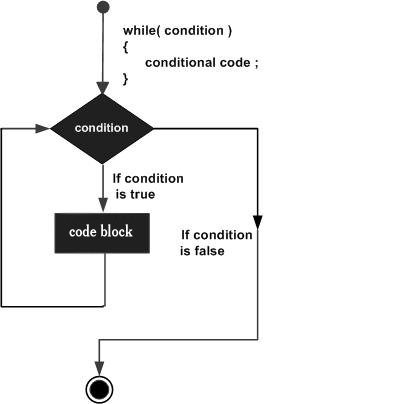Here, key point of the while loop is that the loop might not ever run. When the condition is tested and the result is false, the loop body will be skipped and the first statement after the while loop will be executed.

## Example:

```#include <stdio.h>

int main ()
{
/* local variable definition */
int a = 10;

/* while loop execution */
while( a < 20 )
{
printf("value of a: %d\n", a);
a++;
}

return 0;
}
```

When the above code is compiled and executed, it produces the following result:

```value of a: 10
value of a: 11
value of a: 12
value of a: 13
value of a: 14
value of a: 15
value of a: 16
value of a: 17
value of a: 18
value of a: 19
```

A for loop is a repetition control structure that allows you to efficiently write a loop that needs to execute a specific number of times.

## Syntax:

The syntax of a for loop in C programming language is:

```for ( init; condition; increment )
{
statement(s);
}
```

Here is the flow of control in a for loop:

1. The init step is executed first, and only once. This step allows you to declare and initialize any loop control variables. You are not required to put a statement here, as long as a semicolon appears.

2. Next, the condition is evaluated. If it is true, the body of the loop is executed. If it is false, the body of the loop does not execute and flow of control jumps to the next statement just after the for loop.

3. After the body of the for loop executes, the flow of control jumps back up to the increment statement. This statement allows you to update any loop control variables. This statement can be left blank, as long as a semicolon appears after the condition.

4. The condition is now evaluated again. If it is true, the loop executes and the process repeats itself (body of loop, then increment step, and then again condition). After the condition becomes false, the for loop terminates.

## Flow Diagram: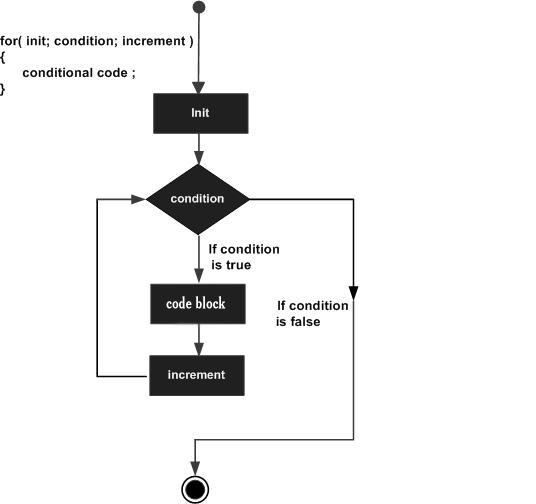## Example:

```#include <stdio.h>

int main ()
{
/* for loop execution */
for( int a = 10; a < 20; a = a + 1 )
{
printf("value of a: %d\n", a);
}

return 0;
}
```

When the above code is compiled and executed, it produces the following result:

```value of a: 10
value of a: 11
value of a: 12
value of a: 13
value of a: 14
value of a: 15
value of a: 16
value of a: 17
value of a: 18
value of a: 19
```

Unlike for and while loops, which test the loop condition at the top of the loop, the do...while loop in C programming language checks its condition at the bottom of the loop.

A do...while loop is similar to a while loop, except that a do...while loop is guaranteed to execute at least one time.

## Syntax:

The syntax of a do...while loop in C programming language is:

```do
{
statement(s);

}while( condition );
```

Notice that the conditional expression appears at the end of the loop, so the statement(s) in the loop execute once before the condition is tested.

If the condition is true, the flow of control jumps back up to do, and the statement(s) in the loop execute again. This process repeats until the given condition becomes false.

## Flow Diagram: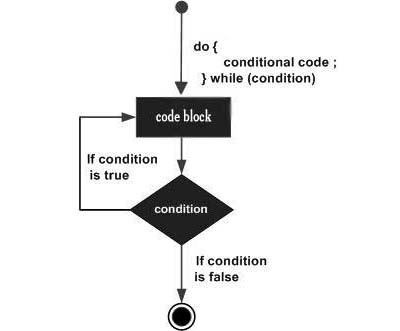## Example:

```#include <stdio.h>

int main ()
{
/* local variable definition */
int a = 10;

/* do loop execution */
do
{
printf("value of a: %d\n", a);
a = a + 1;
}while( a < 20 );

return 0;
}
```

When the above code is compiled and executed, it produces the following result:

```value of a: 10
value of a: 11
value of a: 12
value of a: 13
value of a: 14
value of a: 15
value of a: 16
value of a: 17
value of a: 18
value of a: 19
```

C programming language allows to use one loop inside another loop. Following section shows few examples to illustrate the concept.

## Syntax:

The syntax for a nested for loop statement in C is as follows:

```for ( init; condition; increment )
{
for ( init; condition; increment )
{
statement(s);
}
statement(s);
}
```

The syntax for a nested while loop statement in C programming language is as follows:

```while(condition)
{
while(condition)
{
statement(s);
}
statement(s);
}
```

The syntax for a nested do...while loop statement in C programming language is as follows:

```do
{
statement(s);
do
{
statement(s);
}while( condition );

}while( condition );
```

A final note on loop nesting is that you can put any type of loop inside of any other type of loop. For example, a for loop can be inside a while loop or vice versa.

## Example:

The following program uses a nested for loop to find the prime numbers from 2 to 100:

```#include <stdio.h>

int main ()
{
/* local variable definition */
int i, j;

for(i=2; i<100; i++) {
for(j=2; j <= (i/j); j++)
if(!(i%j)) break; // if factor found, not prime
if(j > (i/j)) printf("%d is prime\n", i);
}

return 0;
}
```

When the above code is compiled and executed, it produces the following result:

```2 is prime
3 is prime
5 is prime
7 is prime
11 is prime
13 is prime
17 is prime
19 is prime
23 is prime
29 is prime
31 is prime
37 is prime
41 is prime
43 is prime
47 is prime
53 is prime
59 is prime
61 is prime
67 is prime
71 is prime
73 is prime
79 is prime
83 is prime
89 is prime
97 is prime
```

The break statement in C programming language has the following two usages:

1. When the break statement is encountered inside a loop, the loop is immediately terminated and program control resumes at the next statement following the loop.

2. It can be used to terminate a case in the switch statement (covered in the next chapter).

If you are using nested loops (i.e., one loop inside another loop), the break statement will stop the execution of the innermost loop and start executing the next line of code after the block.

## Syntax:

The syntax for a break statement in C is as follows:

```break;
```

## Flow Diagram: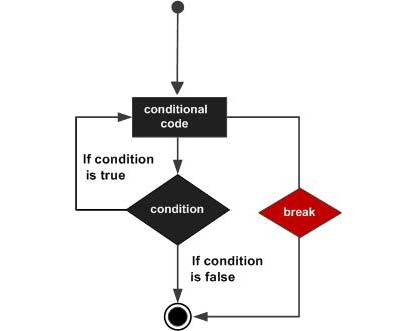## Example:

```#include <stdio.h>

int main ()
{
/* local variable definition */
int a = 10;

/* while loop execution */
while( a < 20 )
{
printf("value of a: %d\n", a);
a++;
if( a > 15)
{
/* terminate the loop using break statement */
break;
}
}

return 0;
}
```

When the above code is compiled and executed, it produces the following result:

```value of a: 10
value of a: 11
value of a: 12
value of a: 13
value of a: 14
value of a: 15
```

A goto statement in C programming language provides an unconditional jump from the goto to a labeled statement in the same function.

NOTE: Use of goto statement is highly discouraged in any programming language because it makes difficult to trace the control flow of a program, making the program hard to understand and hard to modify. Any program that uses a goto can be rewritten so that it doesn't need the goto.

## Syntax:

The syntax for a goto statement in C is as follows:

```goto label;
..
.
label: statement;
```

Here label can be any plain text except C keyword and it can be set anywhere in the C program above or below to goto statement.

## Flow Diagram: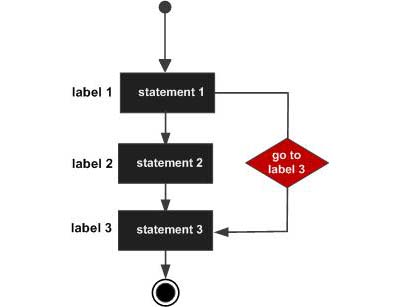## Example:

```#include <stdio.h>

int main ()
{
/* local variable definition */
int a = 10;

/* do loop execution */
LOOP:do
{
if( a == 15)
{
/* skip the iteration */
a = a + 1;
goto LOOP;
}
printf("value of a: %d\n", a);
a++;

}while( a < 20 );

return 0;
}
```

When the above code is compiled and executed, it produces the following result:

```value of a: 10
value of a: 11
value of a: 12
value of a: 13
value of a: 14
value of a: 16
value of a: 17
value of a: 18
value of a: 19
```